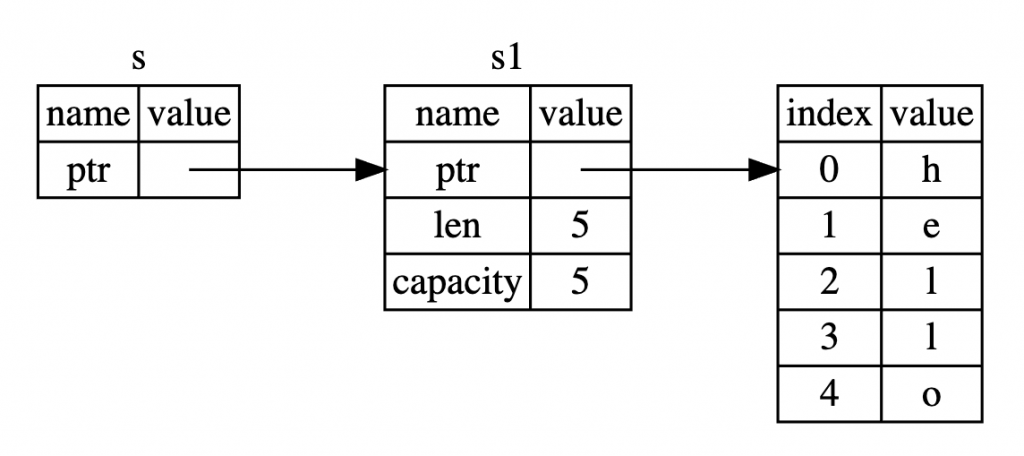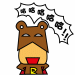#DAY 15
0

## References

``````fn main() {
let s1 = String::from("hello");

let len = calculate_length(&s1); // 傳遞 reference 但是沒有 ownership

println!("The length of '{}' is {}.", s1, len);
}

fn calculate_length(s: &String) -> usize {
s.len()
} // 因為沒有任何 ownership 所以 drop 也不會怎樣
``````

1. 函式的參數多了個 & 的符號
2. s1 還是可以使用 ownership 沒有被轉移

• 第一點 & 的符號就代表這個參數是 reference
• 第二點 reference 讓你可以指到你要的值但是不需要轉移 ownership``````fn main() {
let s = String::from("hello");

unchangeable(&s);
}

fn unchangeable(some_string: &String) {
some_string.push_str(", world");
^^^^^^^^^^^ `some_string` is a `&` reference, so the data it refers to cannot be borrowed as mutable
}
``````

## Mutable References

``````fn main() {
let mut s = String::from("hello");

changeable(&s);

println!("s = {}", s); // s = hello, world
}

fn changeable(some_string: &mut String) {
some_string.push_str(", world");
}
``````

``````fn cause_error() {
let mut s = String::from("hello");
let r1 = &mut s;
let r2 = &mut s;
^^^^^^ second mutable borrow occurs here
println!("{}, {}", r1, r2);
}
``````

• 2 個以上的 mutable reference 同時取得同一個值
• 至少一個 pointer 被用來寫入資料
• 沒有同步訪問數據的機制

``````let mut s = String::from("hello");

let r1 = &s; // no problem
let r2 = &s; // no problem
let r3 = &mut s; // BIG PROBLEM
^^^^^^ mutable borrow occurs here

println!("{}, {}, and {}", r1, r2, r3);
````````````fn this_work() {
let mut s = String::from("hello");

let r1 = &s; // no problem
let r2 = &s; // no problem
println!("{} and {}", r1, r2);
// 在這之後不再使用 r1 和 r2

let r3 = &mut s; // no problem
println!("{}", r3);
}
``````

``````fn this_still_work() {
let mut s = String::from("hello");
let r1 = &mut s;
println!("{}", r1);
// 在這之後不再使用 r1
let r2 = &s;
let r3 = &s;
println!("{} {}", r2, r3);
}
``````

``````fn this_work_too() {
let mut s = String::from("hello");

{
let r1 = &mut s;

} // r1 goes out of scope here, so we can make a new reference with no problems.

let r2 = &mut s;
}
``````## Dangling References

``````fn main() {
let reference_to_nothing = dangling_references();
}

// help: this function's return type contains a borrowed value, but there is no value for it to be borrowed from
fn dangling_references() -> &String {
^ help: consider giving it a 'static lifetime: `&'static`
let s = String::from("hello");

&s
} // s 在這邊已經離開 scope 了但是卻回傳了他的 reference，因此就會造成迷途指標。
``````

``````fn no_dangling_references() -> String {
let s = String::from("hello");

s
}
``````

## 最後一樣有問題歡迎發問